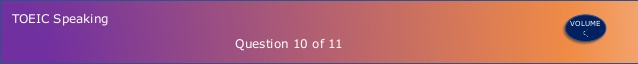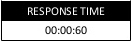## TOEIC Speaking - Question 10

Question 10 - Propose a solution

Questions 10: Propose a solution

Directions: In this part of the test, you will be presented with a problem and asked to propose a solution.

You will have 45 seconds to prepare. Then you will have 60 seconds to speak.

In your response, be sure to
• show that you recognize the problem, and
• propose a way of dealing with the problem.In your response, be sure to
• show that you recognize the problem, and
• propose a way of dealing with the problem.Question

Sample Response 1

Taken from YBM 한국TOEIC위원회

Questions 10: Propose a solution

Directions: In this part of the test, you will be presented with a problem and asked to propose a solution.

You will have 45 seconds to prepare. Then you will have 60 seconds to speak.

In your response, be sure to
• show that you recognize the problem, and
• propose a way of dealing with the problem.In your response, be sure to
• show that you recognize the problem, and
• propose a way of dealing with the problem.Question

Sample Response 1

Taken from YBM 한국TOEIC위원회

Questions 10: Propose a solution

Directions: In this part of the test, you will be presented with a problem and asked to propose a solution.

You will have 45 seconds to prepare. Then you will have 60 seconds to speak.

In your response, be sure to
• show that you recognize the problem, and
• propose a way of dealing with the problem.In your response, be sure to
• show that you recognize the problem, and
• propose a way of dealing with the problem.Question

Sample Response 1

Taken from YBM 한국TOEIC위원회

Questions 10: Propose a solution

Directions: In this part of the test, you will be presented with a problem and asked to propose a solution.

You will have 45 seconds to prepare. Then you will have 60 seconds to speak.

In your response, be sure to
• show that you recognize the problem, and
• propose a way of dealing with the problem.In your response, be sure to
• show that you recognize the problem, and
• propose a way of dealing with the problem.Question

Sample Response 1

Taken from YBM 한국TOEIC위원회

Questions 10: Propose a solution

Directions: In this part of the test, you will be presented with a problem and asked to propose a solution.

You will have 45 seconds to prepare. Then you will have 60 seconds to speak.

In your response, be sure to
• show that you recognize the problem, and
• propose a way of dealing with the problem.In your response, be sure to
• show that you recognize the problem, and
• propose a way of dealing with the problem.Question

Taken from ETS Tactics for TOEIC Speaking and Writing

Questions 10: Propose a solution

Directions: In this part of the test, you will be presented with a problem and asked to propose a solution.

You will have 45 seconds to prepare. Then you will have 60 seconds to speak.

In your response, be sure to
• show that you recognize the problem, and
• propose a way of dealing with the problem.In your response, be sure to
• show that you recognize the problem, and
• propose a way of dealing with the problem.Question

Taken from ETS Tactics for TOEIC Speaking and Writing

Questions 10: Propose a solution

Directions: In this part of the test, you will be presented with a problem and asked to propose a solution.

You will have 45 seconds to prepare. Then you will have 60 seconds to speak.

In your response, be sure to
• show that you recognize the problem, and
• propose a way of dealing with the problem.In your response, be sure to
• show that you recognize the problem, and
• propose a way of dealing with the problem.Question

Taken from ETS Tactics for TOEIC Speaking and Writing

Questions 10: Propose a solution

Directions: In this part of the test, you will be presented with a problem and asked to propose a solution.

You will have 45 seconds to prepare. Then you will have 60 seconds to speak.

In your response, be sure to
• show that you recognize the problem, and
• propose a way of dealing with the problem.In your response, be sure to
• show that you recognize the problem, and
• propose a way of dealing with the problem.Question

Taken from ETS Tactics for TOEIC Speaking and Writing

Questions 10: Propose a solution

Directions: In this part of the test, you will be presented with a problem and asked to propose a solution.

You will have 45 seconds to prepare. Then you will have 60 seconds to speak.

In your response, be sure to
• show that you recognize the problem, and
• propose a way of dealing with the problem.In your response, be sure to
• show that you recognize the problem, and
• propose a way of dealing with the problem.Question

Taken from ETS Tactics for TOEIC Speaking and Writing

Questions 10: Propose a solution

Directions: In this part of the test, you will be presented with a problem and asked to propose a solution.

You will have 45 seconds to prepare. Then you will have 60 seconds to speak.

In your response, be sure to
• show that you recognize the problem, and
• propose a way of dealing with the problem.In your response, be sure to
• show that you recognize the problem, and
• propose a way of dealing with the problem.Question

Taken from ETS Tactics for TOEIC Speaking and Writing

Questions 10: Propose a solution

Directions: In this part of the test, you will be presented with a problem and asked to propose a solution.

You will have 45 seconds to prepare. Then you will have 60 seconds to speak.

In your response, be sure to
• show that you recognize the problem, and
• propose a way of dealing with the problem.In your response, be sure to
• show that you recognize the problem, and
• propose a way of dealing with the problem.Question

Taken from ETS Tactics for TOEIC Speaking and Writing

Questions 10: Propose a solution

Directions: In this part of the test, you will be presented with a problem and asked to propose a solution.

You will have 45 seconds to prepare. Then you will have 60 seconds to speak.

In your response, be sure to
• show that you recognize the problem, and
• propose a way of dealing with the problem.In your response, be sure to
• show that you recognize the problem, and
• propose a way of dealing with the problem.Question

Taken from ETS Tactics for TOEIC Speaking and Writing